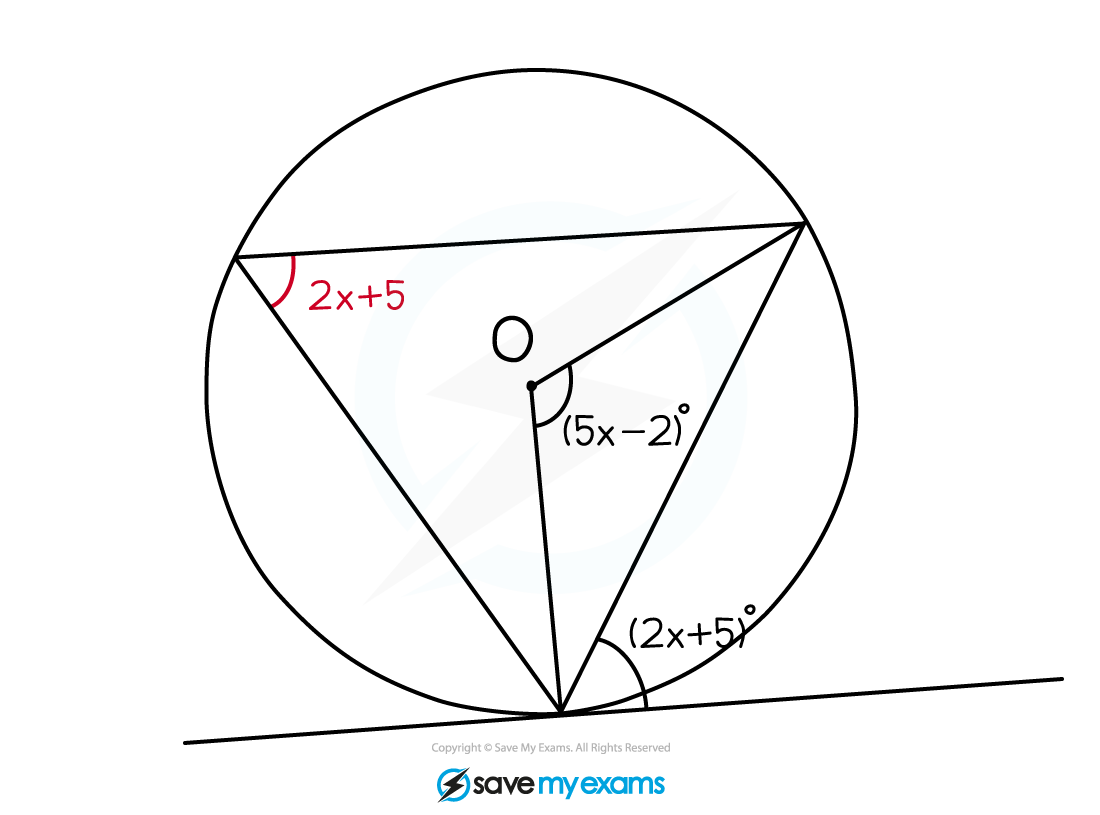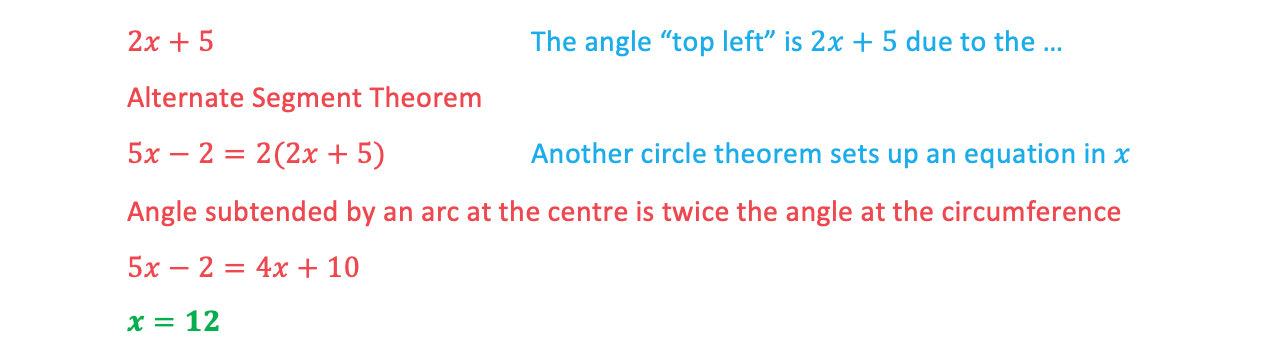# Edexcel IGCSE Maths 复习笔记 4.6.4 Circle Theorems - Alternate Segment

Edexcel IGCSE Maths 复习笔记 4.6.4 Circle Theorems - Alternate Segment

#### What you need to know

1. Alternate Segment Theorem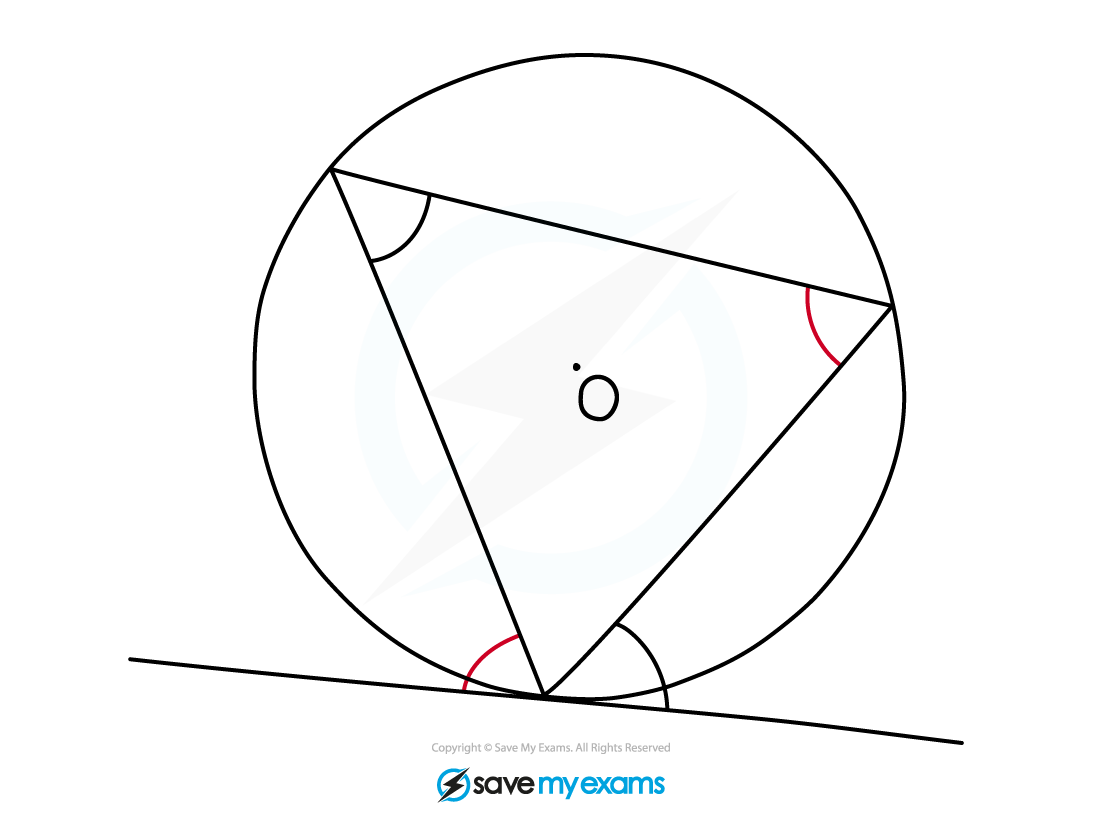• The Alternate Segment Theorem states that the angle between a chord and a tangent is equal to the angle in the alternate segment
• You can spot this circle theorem by looking for a “cyclic triangle”

ie. all 3 vertices lie on the circumference) but one vertex lies on a tangent – look for where 2 chords meet a tangent

#### Exam Tip

Questions often ask for “reasons” – aim to quote an angle fact or circle theorem for every angle you find, not just one for the final answer.

#### Worked Example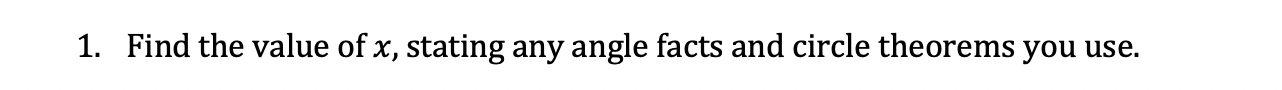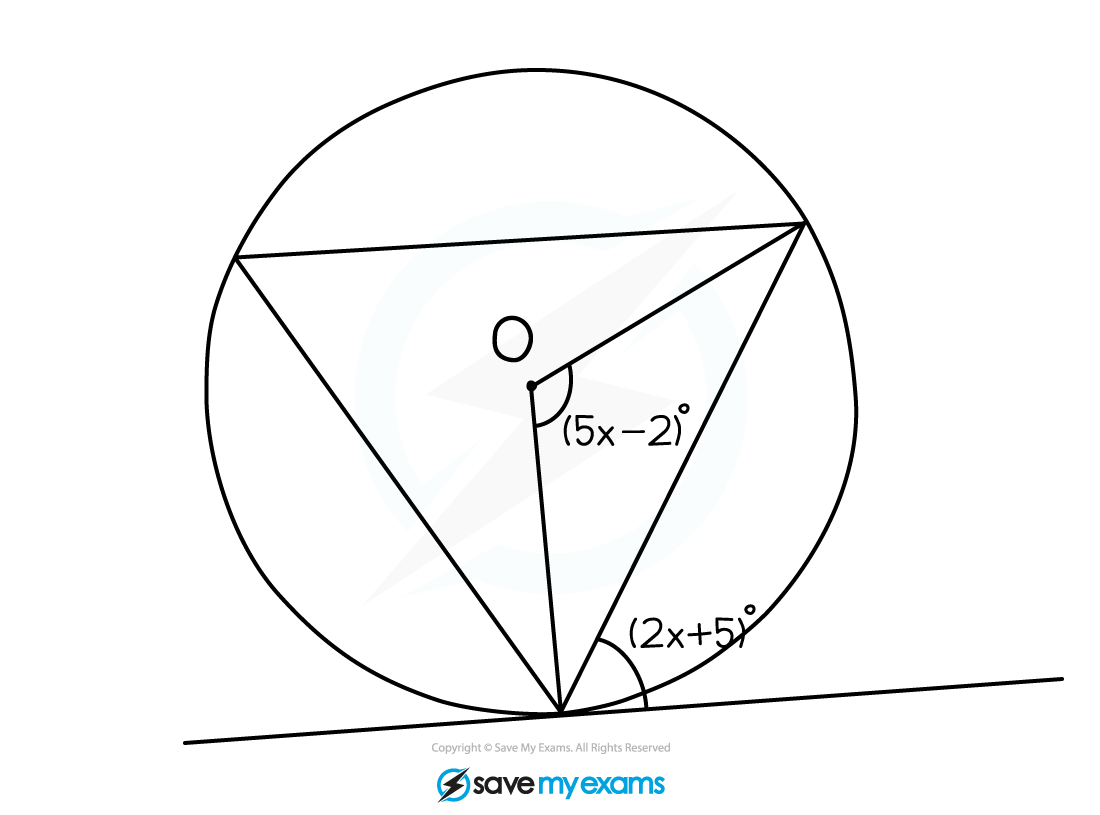#### Worked Example